Inverse of two matrices and verifying properties

Chapter 4 Class 12 Determinants
Concept wise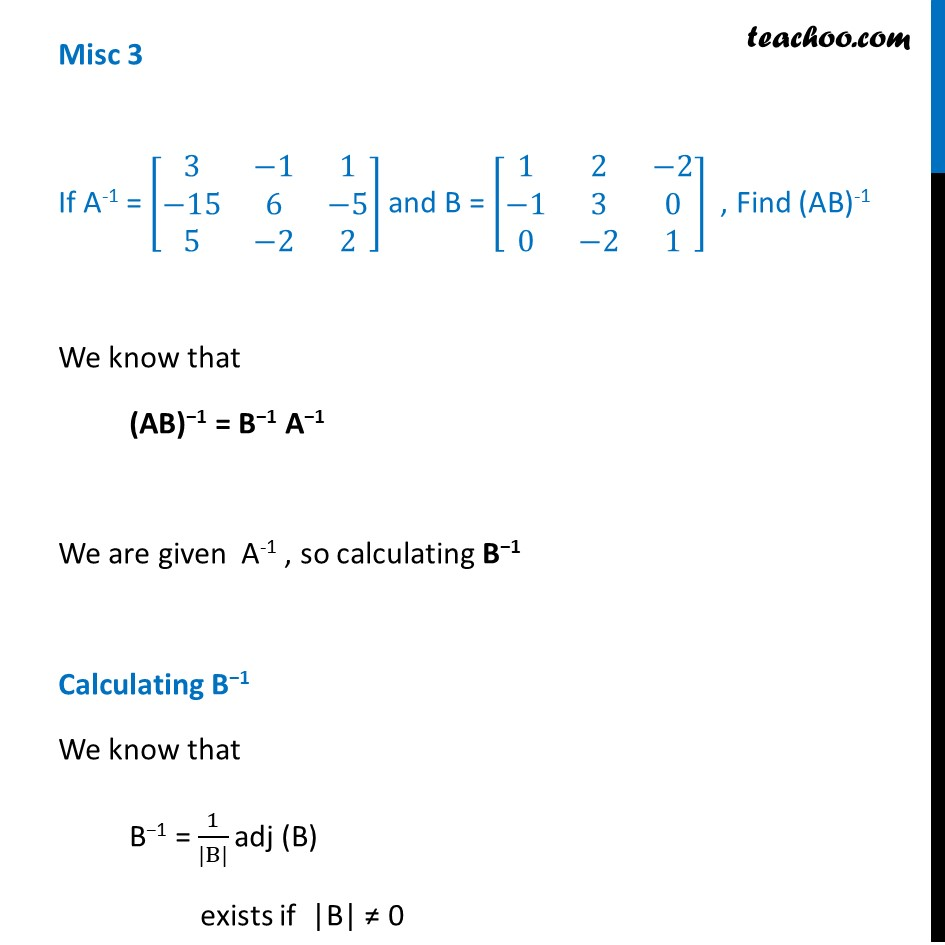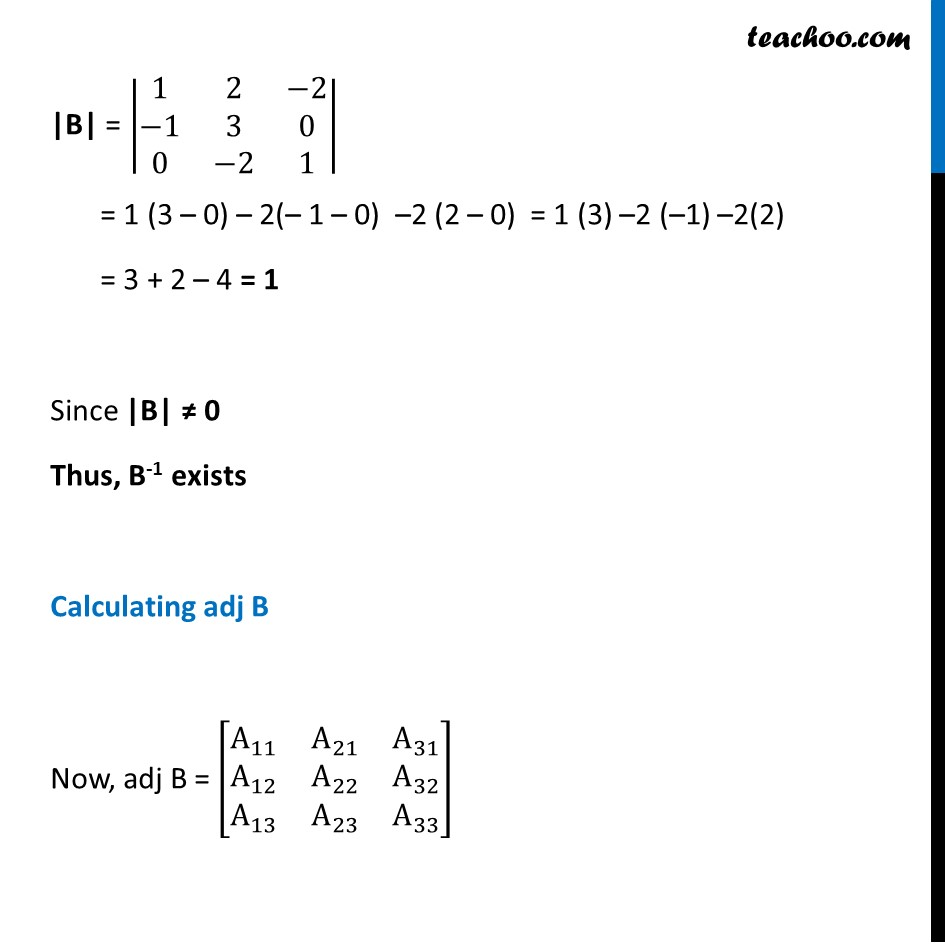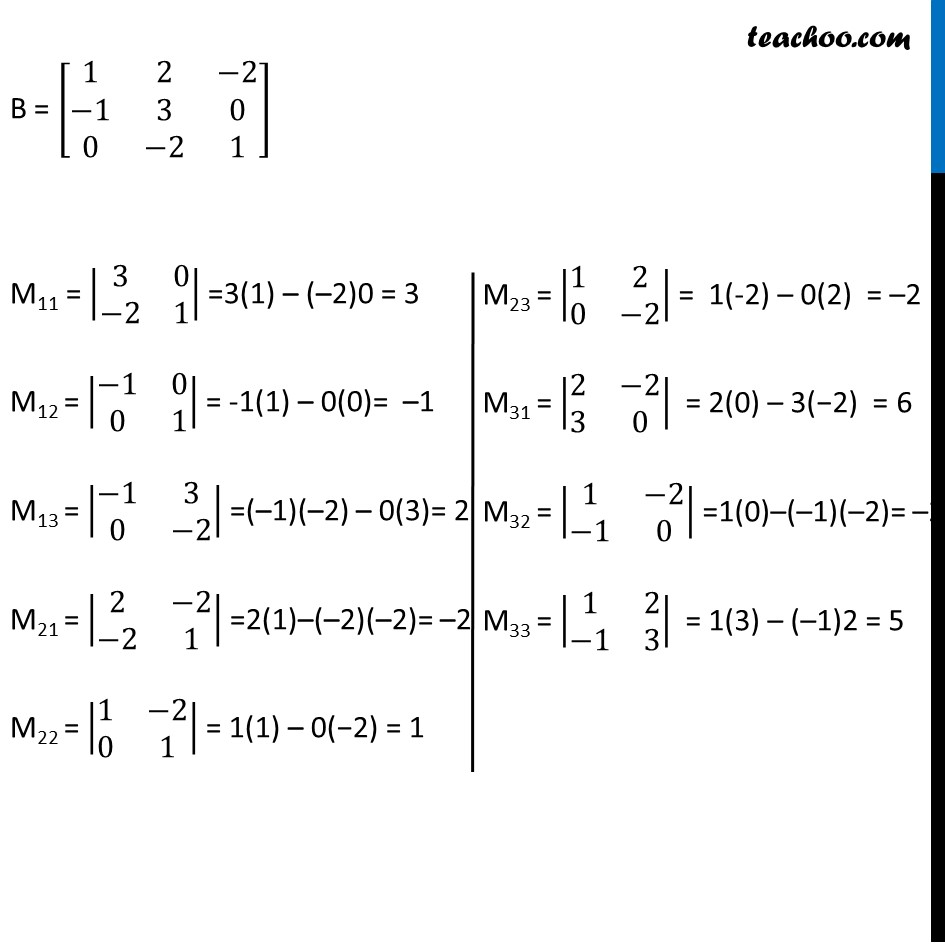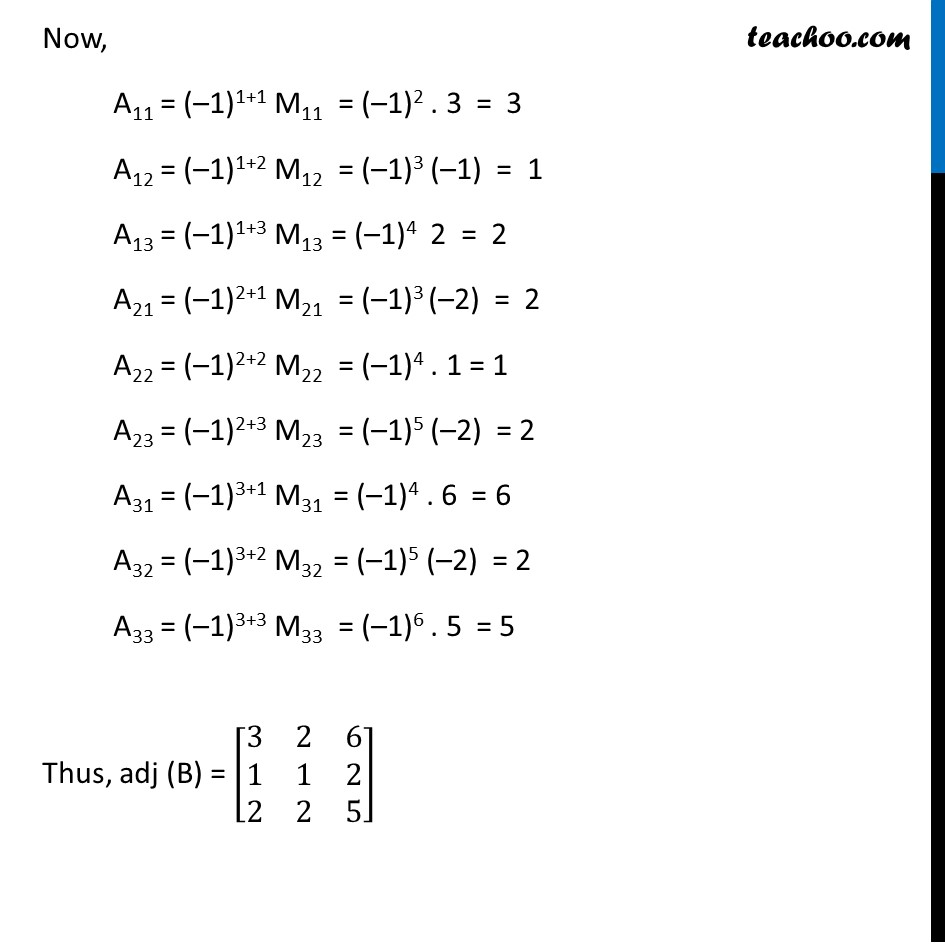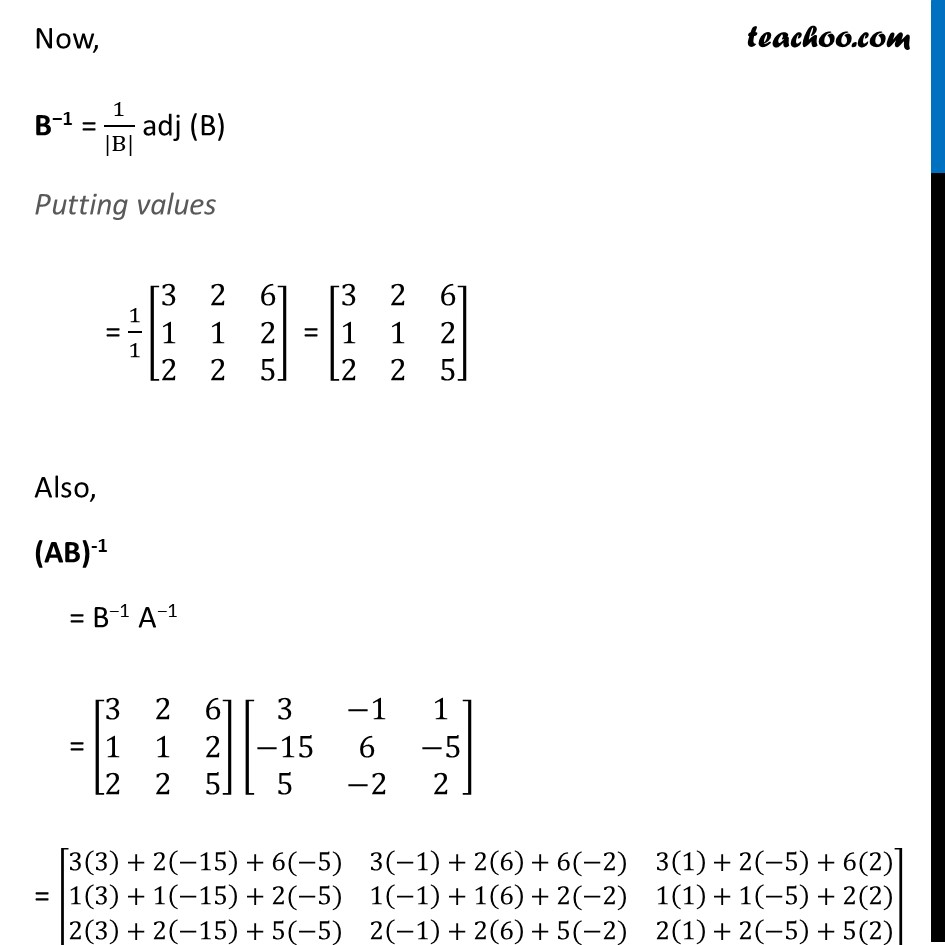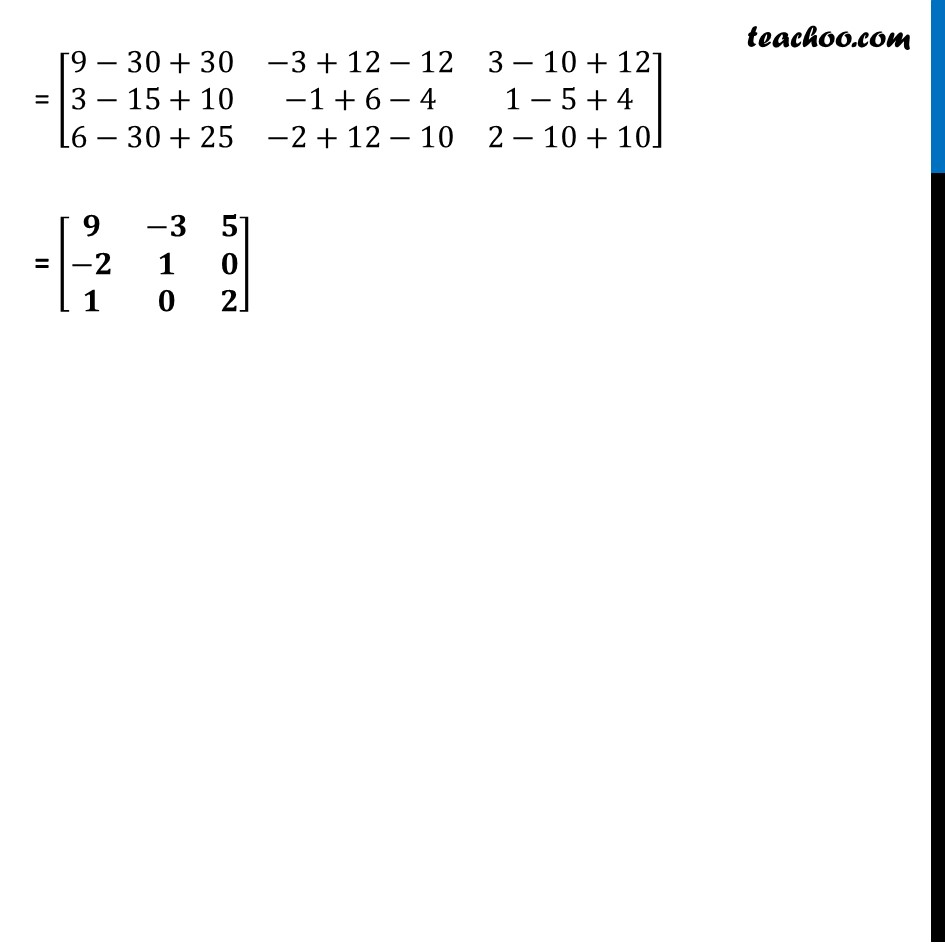Learn in your speed, with individual attention - Teachoo Maths 1-on-1 Class

### Transcript

Misc 3 If A-1 = [■8(3&−1&1@−15&6&−5@5&−2&2)] and B = [■8(1&2&−2@−1&3&0@0&−2&1)] , Find (AB)-1 We know that (AB)−1 = B−1 A−1 We are given A-1 , so calculating B−1 Calculating B−1 We know that B−1 = 1/(|B|) adj (B) exists if |B| ≠ 0 |B| = |■8(1&2&−2@−1&3&0@0&−2&1)| = 1 (3 – 0) – 2(– 1 – 0) –2 (2 – 0) = 1 (3) –2 (–1) –2(2) = 3 + 2 – 4 = 1 Since |B| ≠ 0 Thus, B-1 exists Calculating adj B Now, adj B = [■8(A_11&A_21&A_31@A_12&A_22&A_32@A_13&A_23&A_33 )] B = [■8(1&2&−2@−1&3&0@0&−2&1)] M11 = |■8(3&0@−2&1)| =3(1) – (–2)0 = 3 M12 = |■8(−1&0@0&1)| = -1(1) – 0(0)= –1 M13 = |■8(−1&3@0&−2)| =(–1)(–2) – 0(3)= 2 M21 = |■8(2&−2@−2&1)| =2(1)–(–2)(–2)= –2 M22 = |■8(1&−2@0&1)| = 1(1) – 0(−2) = 1 M23 = |■8(1&2@0&−2)| = 1(-2) – 0(2) = –2 M31 = |■8(2&−2@3&0)| = 2(0) – 3(−2) = 6 M32 = |■8(1&−2@−1&0)| =1(0)–(–1)(–2)= –2 M33 = |■8(1&2@−1&3)| = 1(3) – (–1)2 = 5 Now, A11 = (–1)1+1 M11 = (–1)2 . 3 = 3 A12 = (–1)1+2 M12 = (–1)3 (–1) = 1 A13 = (–1)1+3 M13 = (–1)4 2 = 2 A21 = (–1)2+1 M21 = (–1)3 (–2) = 2 A22 = (–1)2+2 M22 = (–1)4 . 1 = 1 A23 = (–1)2+3 M23 = (–1)5 (–2) = 2 A31 = (–1)3+1 M31 = (–1)4 . 6 = 6 A32 = (–1)3+2 M32 = (–1)5 (–2) = 2 A33 = (–1)3+3 M33 = (–1)6 . 5 = 5 Thus, adj (B) = [■8(3&2&6@1&1&2@2&2&5)] Now, B−1 = 1/(|B|) adj (B) Putting values = 1/1 [■8(3&2&6@1&1&2@2&2&5)] = [■8(3&2&6@1&1&2@2&2&5)] Also, (AB)-1 = B−1 A−1 = [■8(3&2&6@1&1&2@2&2&5)] [■8(3&−1&1@−15&6&−5@5&−2&2)] = [■8(3(3)+2(⤶7−15)+6(−5)&3(−1)+2(6)+6(−2)&3(1)+2(−5)+6(2)@1(3)+1(⤶7−15)+2(−5)&1(−1)+1(6)+2(−2)&1(1)+1(−5)+2(2)@2(3)+2(⤶7−15)+5(−5)&2(−1)+2(6)+5(−2)&2(1)+2(−5)+5(2))] = [■8(9−30+30&−3+12−12&3−10+12@3−15+10&−1+6−4&1−5+4@6−30+25&−2+12−10&2−10+10)] = [■8(𝟗&−𝟑&𝟓@−𝟐&𝟏&𝟎@𝟏&𝟎&𝟐)]